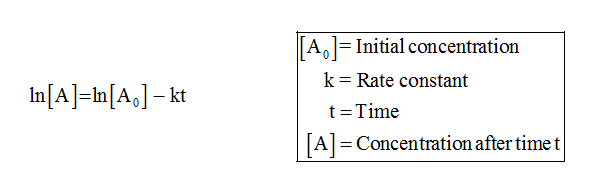# The first-order decomposition of N2O at 1000 K has a rate constant of 0.76 s-1. If the initial concentration of N2O is 10.9 M, what is the concentration of N2O after 9.6 s?

Question
26 views

The first-order decomposition of N2O at 1000 K has a rate constant of 0.76 s-1. If the initial concentration of N2O is 10.9 M, what is the concentration of N2O after 9.6 s?

check_circle

Step 1

Concentration of N2O after 9.6 s can be determined using the given formula,...

### Want to see the full answer?

See Solution

#### Want to see this answer and more?

Solutions are written by subject experts who are available 24/7. Questions are typically answered within 1 hour.*

See Solution
*Response times may vary by subject and question.
Tagged in

### Chemistry